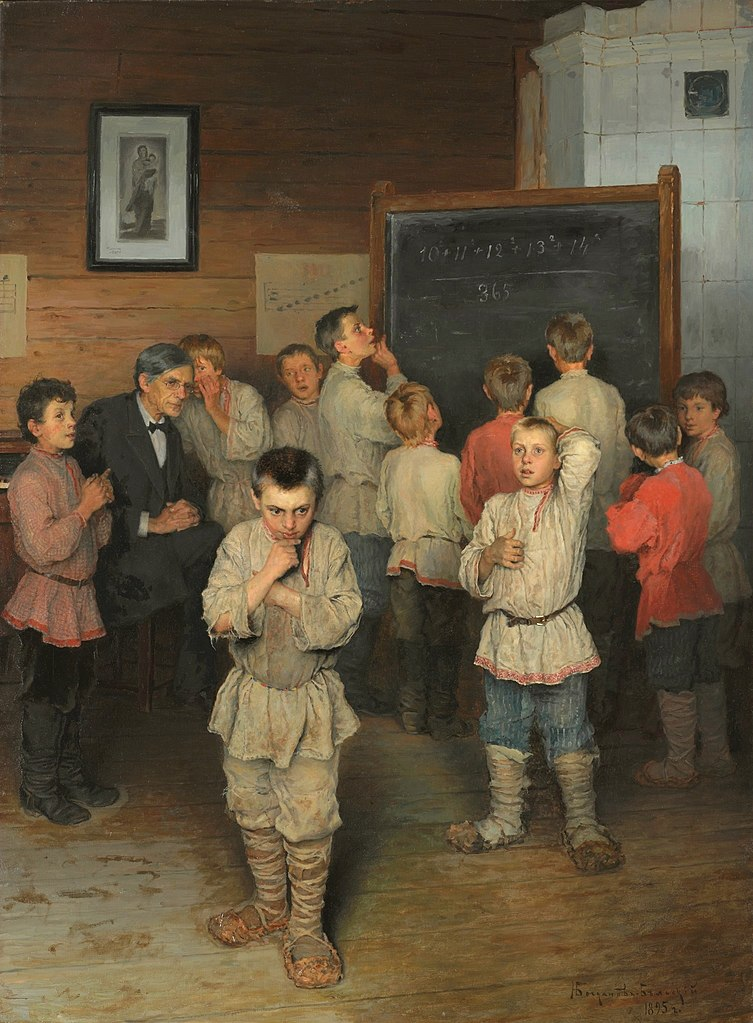# Extra CreditThe boys in Nikolay Bogdanov-Belsky’s 1895 painting Mental Arithmetic are having a difficult time solving the problem on the board:$\displaystyle \frac{10^{2} + 11^{2} + 12^{2} + 13^{2} + 14^{2}}{365}$

As it happens, there’s a simple solution: Both (102 + 112 + 122) and (132 + 142) are equal to 365, so the answer is simply (365 + 365) / 365, or 2. They’ll figure it out.